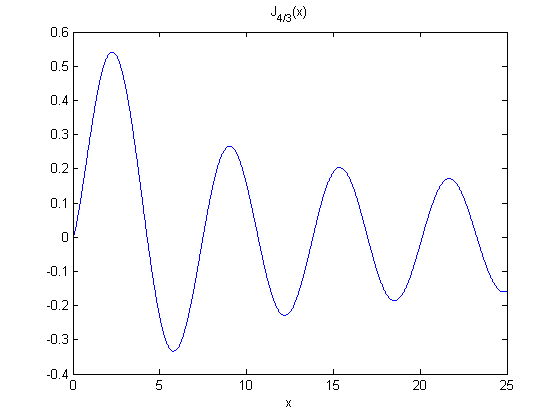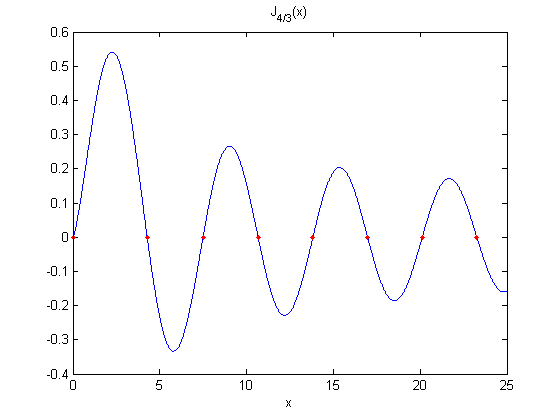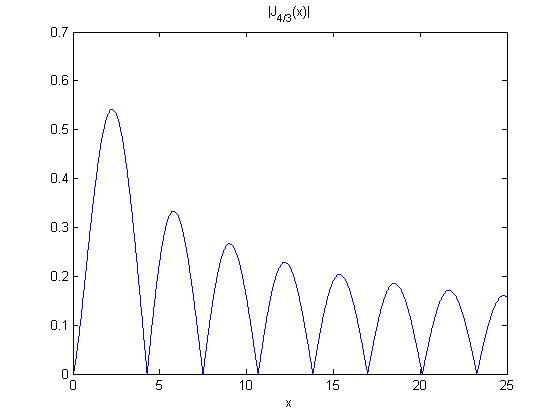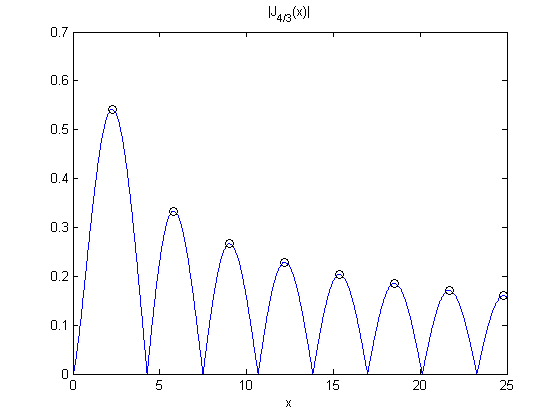# CHEBFUN, Roots

The ROOTS function in Chebfun is one of its most powerful features. This is the second part of my series on Chebfun. Part one is here.

### Contents

#### Roots versus zeros

Before I wrote this blog I tried to make a distinction between the mathematical terms "roots" and "zeros". As far as I was concerned, equations had "roots" while functions had "zeros". The roots of the equation $x^3 = 2x + 5$ were the zeros of the polynomial $x^3 - 2x - 5$. But now I've decided to stop trying to make that distinction. The MATLAB function roots finds all of the roots of a polynomial. No equation or interval or starting approximation is involved. But roots applies only to polynomials. The MATLAB function fzero finds only one zero of a function, not an equation, near a specified starting value or, better yet, in a specified interval. To find many zeros you have to call fzero repeatedly with carefully chosen starting values. So MATLAB does not make the rigorous distinction between roots and zeros that I used to desire. Chebfun has a very powerful and versatile function named roots. A chebfun is a highly accurate polynomial approximation to a smooth function, so the roots of a chebfun, which are the roots of a polynomial, are usually excellent approximations to the zeros of the underlying function. And Chebfun's roots will find all of them in the interval of definition, not just one. So, Chebfun has helped convince me to stop trying to distinguish between "roots" and "zeros".

#### Companion and colleague matrices.

When I was writing the first MATLAB years ago I was focused on matrix computation and was concerned about code size and memory space, so when I added roots for polynomials I simply formed the companion matrix and found its eigenvalues. That was a novel approach for polynomial root finding at the time, but it has proved effective and is still used by the MATLAB roots function today. Chebfun continues that approach by employing colleague matrices for the Chebfun roots function. I had never heard of colleague matrices until the Oxford workshop a few weeks ago. The eigenvalues of the colleague matrix associated with a Chebyshev polynomial provide the roots of that polynomial in the same way that the eigenvalues of the companion matrix associated with a monic polynomial provide its roots.

#### Bessel function example

Let's pursue an example involving a fractional order Bessel function. Because of the fractional order, this function has a mild singularity at the origin, so we should turn on Chebfun's splitting option.
help splitting
splitting on

 SPLITTING   CHEBFUN splitting option
SPLITTING ON allows the Chebfun constructor to split the interval by a
process of automatic subdivision and edge detection.  This option is
recommended when working with functions with singularities.


format compact
nu = 4/3
a = 25
J = chebfun(@(x) besselj(nu,x),[0 a]);
lengthJ = length(J)
plot(J)
xlabel('x')
title('J_{4/3}(x)')

nu =
1.3333
a =
25
lengthJ =
385Here are all of our example function's zeros in the interval.
r = roots(J)
hold on
plot(r,0*r,'r.')
hold off

r =
0.0000
4.2753
7.4909
10.6624
13.8202
16.9720
20.1206
23.2673Without Chebfun, that would have required apriori knowledge about the number and approximate location of the zeros in the interval and then a for loop around calls to fzero.

#### Hidden uses

Chebfun frequently invokes roots where it might not be obvious. For example, finding the absolute value of a function involves finding its zeros.
plot(abs(J))
xlabel('x')
title('|J_{4/3}(x)|')Let's find the zeros of the first derivative so we can plot circles at the local maxima. The derivative is computed by differentiating the Chebyshev polynomial, not by symbolic differentiation of the Bessel function.
z = roots(diff(J))
hold on
plot(z,abs(J(z)),'ko')
hold off

z =
2.2578
5.7993
9.0218
12.2005
15.3637
18.5194
21.6709
24.8200Published with MATLAB® R2012b

|

### コメント

コメントを残すには、ここ をクリックして MathWorks アカウントにサインインするか新しい MathWorks アカウントを作成します。